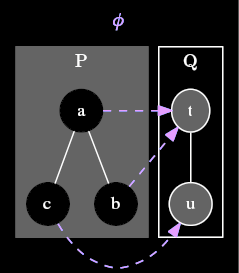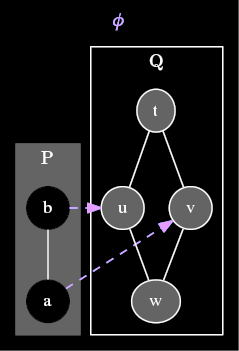# Monotone function

Let $$\langle P, \leq_P \rangle$$ and $$\langle Q, \leq_Q \rangle$$ be posets. Then a function $$\phi : P \rightarrow Q$$ is said to be monotone (alternatively, order-preserving) if for all $$s, t \in P$$, $$s \le_P t$$ implies $$\phi(s) \le_Q \phi(t)$$.

## Positive examplecomment: dot source:

digraph G { node = 0.1, height = 0.1 rankdir = BT; rank = same; compound = true; fontname=”MathJax_Main”;

subgraph cluster_P { node style=filled,color=white; edge = “none”; style = filled; color = lightgrey; fontcolor = black; label = “P”; labelloc = b; b → a; c → a;

} subgraph cluster_Q { node style=filled; edge = “none”; color = black; fontcolor = black; label= “Q”; labelloc = b; u → t; } edge = blue, style = dashed fontcolor = blue; label = “φ”;
labelloc = t; b → t = false; a → t = false; c → u = false; }

<div>

Here is an example of a monotone map $$\phi$$ from a poset $$P$$ to another poset $$Q$$. Since $$\le_P$$ has two comparable pairs of elements, $$(c,a)$$ and $$(b,a)$$, there are two constraints that $$\phi$$ must satisfy to be considered monotone. Since $$c \leq_P a$$, we need $$\phi(c) = u \leq_Q t = \phi(a)$$. This is, in fact, the case. Also, since $$b \leq_P a$$, we need $$\phi(b) = t \leq_Q t = \phi(a)$$. This is also true.

## Negative examplecomment: dot source:

digraph G { node = 0.1, height = 0.1 rankdir = BT; rank = same; compound = true; fontname=”MathJax_Main”;

subgraph cluster_P { node style=filled,color=white; edge = “none”; style = filled; color = lightgrey; fontcolor = black; label = “P”; labelloc = b; a → b; }

subgraph cluster_Q { node style=filled; edge = “none”; color = black; fontcolor = black; label= “Q”; labelloc = b; w → u; w → v; u → t; v → t; } edge = blue, style = dashed fontcolor = blue; label = “φ”;
labelloc = t; b → u = false; a → v = false; } <div>

Here is an example of another map $$\phi$$ between two other posets $$P$$ and $$Q$$. This map is not monotone, because $$a \leq_P b$$ while $$\phi(a) = v \parallel_Q u = \phi(b)$$.